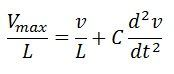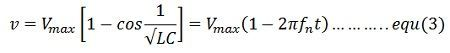# Restriking Transient Voltage

When the current across the contact of the circuit breaker is zero, a high-frequency transient voltage develops in the whole breaker contact and is produced by the sudden distribution of energy between the electric and magnetic field. This transient voltage is called restriking voltage. The voltage appears across the breaker contacts at the moment of final current has a serious influence on the arc extinction process. Under the influence of this voltage, the arc tries to restrike and hence it is named as the restriking voltage.

After the zero current, the arc gets extinguished, if the rate of rising of restriking voltage between the contact is less than the rate at which the dielectric strength of the medium between the contact gains. Immediately after the final current interruption, the voltage that appears across the breaker contacts (transient voltage) superimposed on the power frequency system voltage (recovery voltage).

Considered a simple circuit, having a circuit breaker CB, as shown in the figure below. Let L be the inductance per phase of the system up to the fault point; R be the resistance per phase of the system up to the fault point, and C be the capacitance of the circuit.When the fault occurs in the system under fault condition the contacts of the breaker are open, and the capacitance C is short-circuited by the fault, and the short circuit current is limited by the resistance and the inductance.

When the breaker contacts are opened, and the arc certainly quenches at some current zero, a voltage v is suddenly applied across the capacitor and therefore across the circuit breaker contacts. The current i which would flow to the fault is not injected in the capacitor and inductor. ThusAssuming Zero time at zero currents when t = 0 and the value of current and voltage before opening of circuit breaker is expressed asThe solution of the standard equation isFrom the equation,The above expression is for restriking voltage where Vmax is the peak value of recovery voltage (phase -to-neutral) t is time is seconds. L is inductance in Henrys, C is the capacitance in farads and v is the restriking voltage in volts. The maximum value of restriking voltage is 2Vmax and occurs at

###Characteristic of Restriking Voltage

The important characteristic of restriking voltage which affects the performance of the circuit breaker is as follows –

Amplitude Factor – It is defined as the ratio of the peak of transient voltage to the peak system frequency voltage.

The rate of Rising of Restriking Voltage – It is defined as the slope of the steepness tangent of the restriking voltage curve. It is expressed in kV/µs. RRRV is directly proportional to the natural frequency. The expression for the restriking voltage is expressed asThe transient voltage vanishes rapidly due to the damping effect of system resistance, and the normal frequency system voltage is established. This voltage across the breakers contact is called recovery voltage.The waveforms of recovery and the restricting voltage are shown in the figure above. After the current zero, the voltage appearing across the breaker contacts is composed of transient restriking voltage and power frequency recovery voltage.

### 5 thoughts on “Restriking Transient Voltage”

1. Kindly help me answer the question below.
In a short-circuit test on a particular circuit breaker,the following readings were obtained,.
i) Time reached re-striking voltage 50000000 seconds
ii)Pick striking voltage 100KV.
Determine the average reverse recovery re-striking voltage and frequency oscillations